## Sunday, November 13, 2016

### If two angles of a triangle are congruent, then the sides opposite these angles are also congruent

Triangle ABC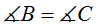Prove

AB = AC

Proof
Drawing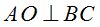See figure 2Figure 2
1:- Given
2: AO = AO - Common
3: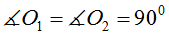- From drawingSee figure 2

4: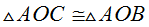- From 1,2,3 - If two angles and a nonincluded side of one triangle are congruent to two angles and a nonincluded side of a second triangle, then the triangles are congruent (AAS)

AB = AB - From 4

## Wednesday, November 9, 2016

### If two sides of a triangle are congruent, then the angles opposite these sides are also congruent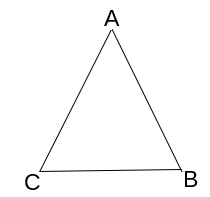Given

Isosceles triangle ABC, AB = AC

ProveProof

1: AB = AC  -  Given
2: Draw angle bisector AO from A to BC
3: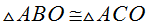The bisector of the vertex angle of an isosceles triangle separates the triangle into two congruent triangles

4:CPCTC (Corresponding parts of congruent triangles are congruent)

## Tuesday, November 8, 2016

### The bisector of the vertex angle of an isosceles triangle separates the triangle into two congruent triangles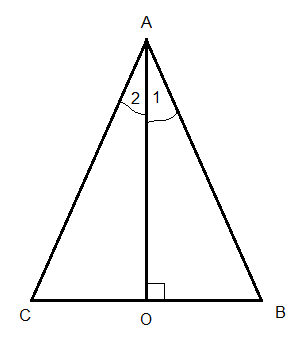Bisector of the vertex angle of an isosceles triangle
Given
Isosceles triangle ABC with AB = AC
AO bisects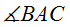,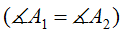Prove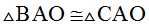Proof

1: AB=AC - Given
2: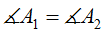- Given

3: AO = AO - Identity- From 1,2,3 - If two sides and the included angle of one triangle are congruent to two sides and the included angle of a second triangle, then the triangles are congruent (SAS)

## Sunday, November 6, 2016

### If two angles and a nonincluded side of one triangle are congruent to two angles and a nonincluded side of a second triangle, then the triangles are congruent (AAS)

Given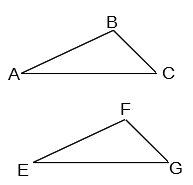Triangles ABC, EFG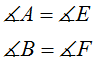AC = EG

Prove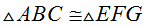Proof
1:- Given
2: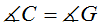- From 1  - If two angles of one triangle are congruent to two angles of another triangle, then the third angles are also congruent

3: AC = EG - Given

4:- From 1,2,3 - If two angles  and the included side of one triangle are congruent to two angles and the included side of a second triangle, then the triangles are congruent (ASA).

## Saturday, November 5, 2016

### If two angles of one triangle are congruent to two angles of another triangle, then the third angles are also congruent.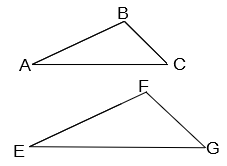Given

Triangles ABC, EFGProveProof
1:- Given

2: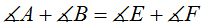- From 1

3: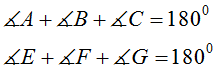- In a triangle, the sum of the measures of the interior angles is 180°

4: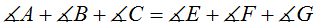- From 3

5: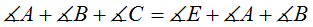- substitute 2 in 4

6:- From 5

## Friday, November 4, 2016

### Corresponding altitudes of congruent triangles are congruentGiven: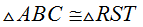Altitudes CD to AB and TV to RS

Prove
CD = TV

Proof
1: AC = RT - correosponding sides in congruent triangles () are equal

2: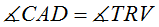- correosponding angles in congruent triangles () are equal

3: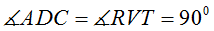- Given (Altitudes CD to AB and TV to RS)

4: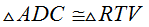- From 1,2,3 AAS - If two angles and a nonincluded side of one triangle are congruent to two angles and a nonincluded side of a second triangle, then the triangles are congruent (AAS)

5: CD = TV - CPCTC - Congruent Parts  Congruent Triangles Congruent

## Friday, October 28, 2016

### The measure of an exterior angle of a triangle equals the sum of the measures of the two nonadjacent interior angles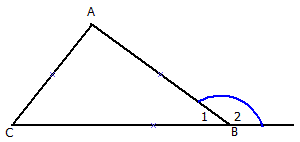Exterior angle of a triangle
Given
Triangle ABC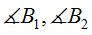- Two adjacent angles form a straight line (BC)

Prove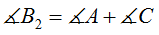Proof
1: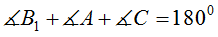- In a triangle, the sum of the measures of the interior angles is 180°

2: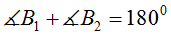- If the exterior sides of two adjacent angles form a straight line, these angles are supplementary- From 1 and 2